Data exploration with ggplot

Set working directory

setwd("C:/Users/lisanjie2/Desktop/TEACHING/1_STATS_CalU/1_STAT_CalU_2016_by_NLB/1_STAT_Duq_2017/HOMEWORK/Duq_Biostats_Homework_4_Milk_regression/homework4_RMD_vs2")

Load data cleaned in previous tutorial

In the PCA tutorial we determined that mammals from Australia are outliers in some respects. We’ll make a new column to indicate which species these are.

i.weirdos <- which(dat$order %in% c("Monotremata" #monotremes ,"Diprotodontia" #marsupials ,"Didelphimorphia"#opossums ,"Dasyuromorphia"#tasmanian devil) ,"Peramelemorphia" #bandicoots )) dat$Australia <- "other"

dat$Australia[i.weirdos] <- "Australia" Subset data for further analyses • We won’t need all of the columns for our regression analysis, so I’ll subset it using “column indexing” • I’ll make an “index” object (i.columns) to hold the names of the columns I want to use • I’ll use that index in a set of square brackets to subset the data • “dat[ , i.columns]” Define columns of interest i.columns <- c("fat", "protein.num", "sugar.num", "energy.num", "mass.female", "gestation.month", "lacatation.months", "mass.litter", "repro.output", "dev.stage.at.birth", "diet","arid", "biome", "Australia", "order", "family", "spp") Subset data using column names dat2 <- dat[ , i.columns] Look at subset dim(dat2) ##  130 17 summary(dat2) ## fat protein.num sugar.num energy.num ## Min. : 0.20 Min. : 1.100 Min. : 0.02 Min. :0.360 ## 1st Qu.: 4.65 1st Qu.: 4.125 1st Qu.: 3.00 1st Qu.:0.965 ## Median : 8.55 Median : 6.750 Median : 4.70 Median :1.365 ## Mean :13.99 Mean : 6.673 Mean : 4.94 Mean :1.680 ## 3rd Qu.:16.82 3rd Qu.: 9.200 3rd Qu.: 6.60 3rd Qu.:2.045 ## Max. :61.10 Max. :15.800 Max. :14.00 Max. :5.890 ## NA's :16 NA's :16 ## mass.female gestation.month lacatation.months ## Min. : 8 Min. : 0.400 Min. : 0.300 ## 1st Qu.: 857 1st Qu.: 1.405 1st Qu.: 1.625 ## Median : 5716 Median : 5.000 Median : 4.500 ## Mean : 2229475 Mean : 5.624 Mean : 6.092 ## 3rd Qu.: 107500 3rd Qu.: 8.365 3rd Qu.: 8.225 ## Max. :170000000 Max. :21.460 Max. :42.000 ## ## mass.litter repro.output dev.stage.at.birth diet ## Min. : 0.3 Min. :0.00003 Min. :0.000 carnivore:32 ## 1st Qu.: 42.0 1st Qu.:0.04000 1st Qu.:1.000 herbivore:61 ## Median : 423.5 Median :0.08000 Median :2.000 omnivore :37 ## Mean : 52563.8 Mean :0.10374 Mean :1.831 ## 3rd Qu.: 7038.2 3rd Qu.:0.13750 3rd Qu.:3.000 ## Max. :2272500.0 Max. :0.50000 Max. :4.000 ## ## arid biome Australia order ## no :91 aquatic : 22 Length:130 Artiodactyla :23 ## yes:39 terrestrial:108 Class :character Carnivora :23 ## Mode :character Primates :22 ## Rodentia :17 ## Chiroptera :10 ## Diprotodontia:10 ## (Other) :25 ## family spp ## Bovidae :13 Acomys cahirinus : 1 ## Cercopithecidae: 8 Alces alces : 1 ## Cervidae : 7 Aloutta palliata : 1 ## Muridae : 7 Aloutta seniculus : 1 ## Otariidae : 7 Arctocephalus australis: 1 ## Phocidae : 7 Arctocephalus gazella : 1 ## (Other) :81 (Other) :124 Data exploration in ggplot2 • Our focal response (y) variable will “fat”, the percentage of fat in each species of mammal’s milk • We’ll use female body size to take a look at the data Plot raw data in base graphics #plot(fat ~ mass.female, data = dat2) Plot raw data in ggplot • We’ll use the ggplot function qplot() • Note that ggplot does NOT use R’s formula notation library(ggplot2) qplot(y = fat, x = mass.female, data = dat2)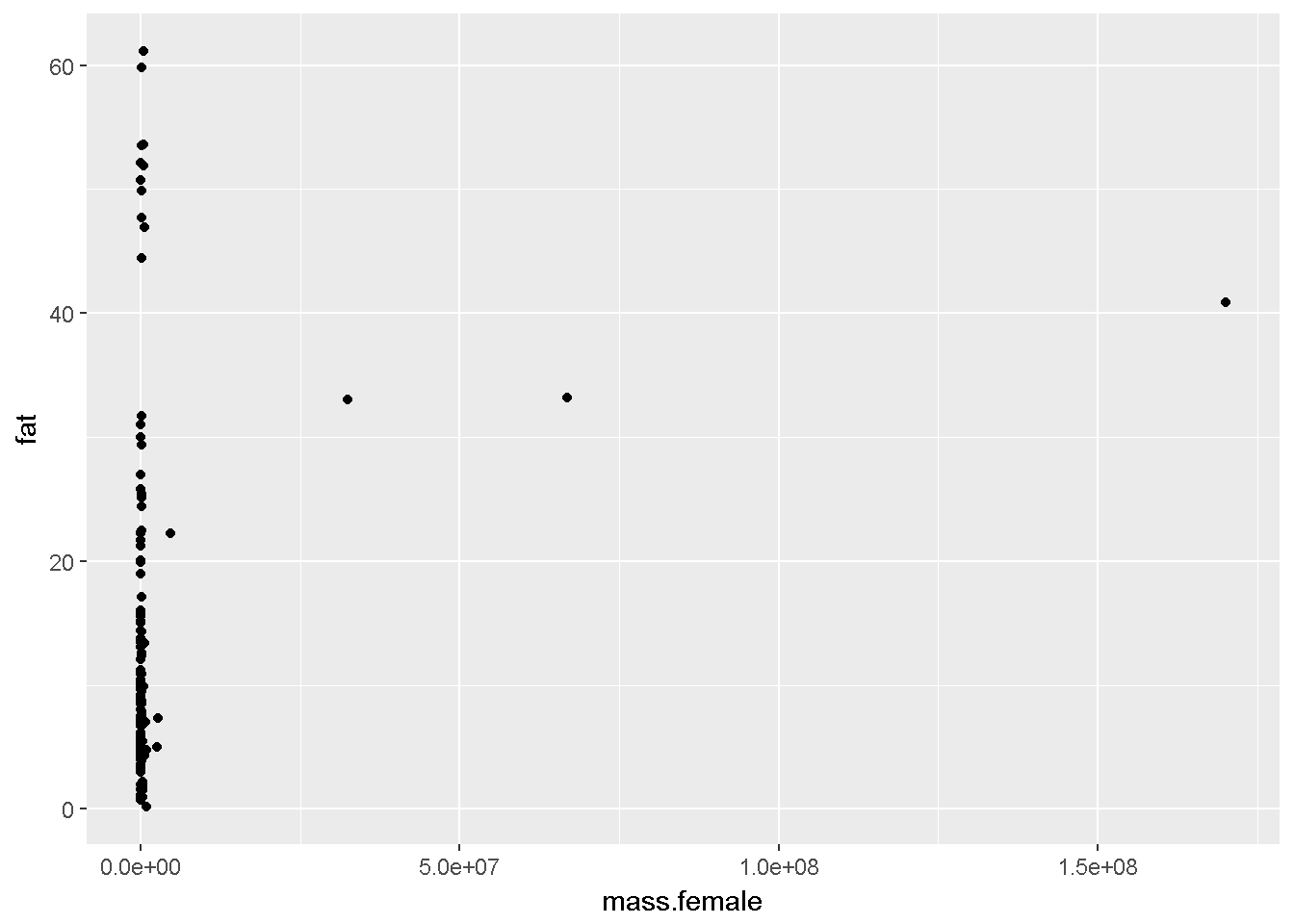Log transform x variable The whales really throw off the x-axis! qplot(y = fat, x = log10(mass.female), data = dat2)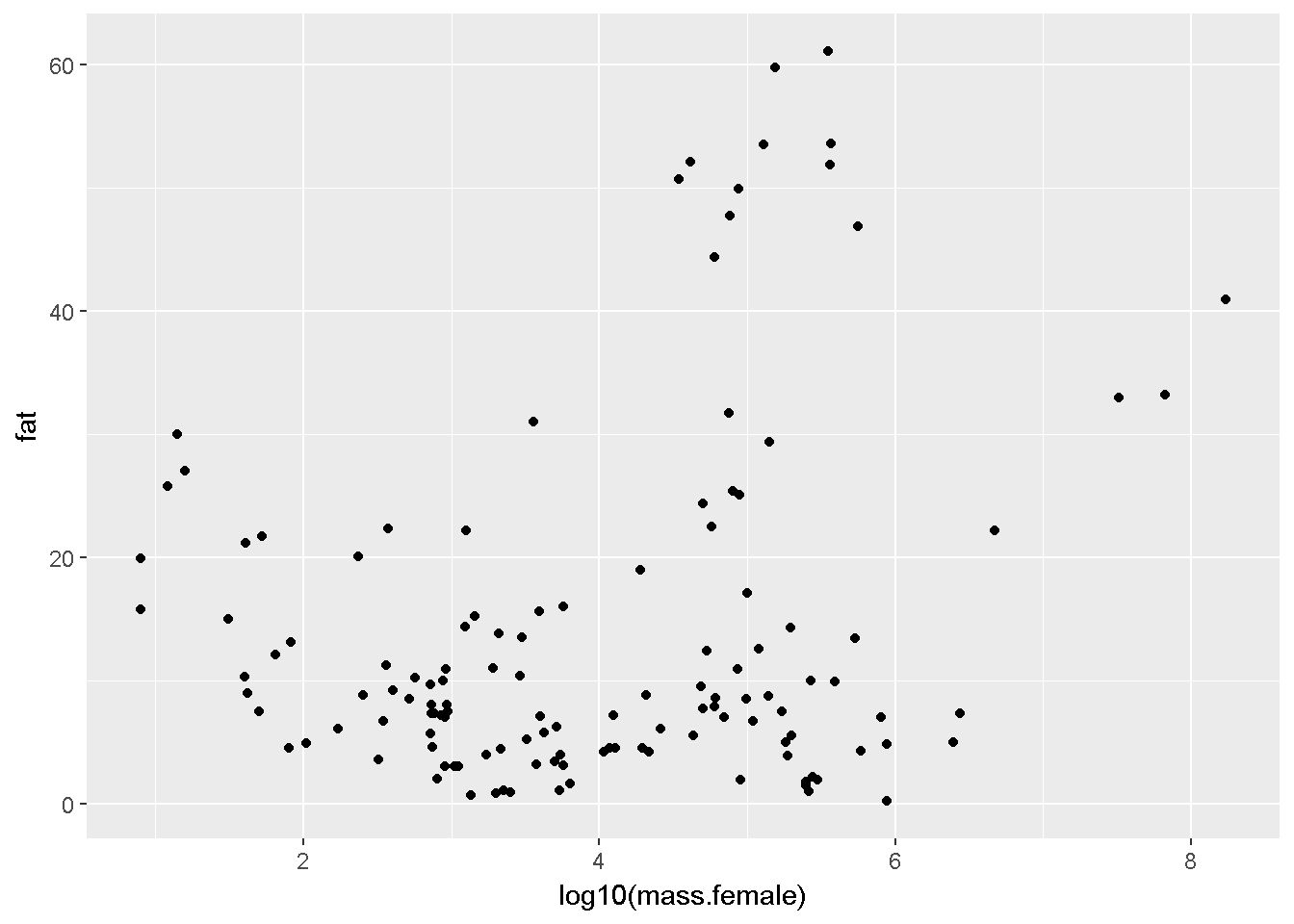Log transform x and y variable • Authors state that “All continuous variables were log10-transformed prior to analysis.” • Log10 transformation is common in biology in general, and especialy when things like body size are being considered. qplot(y = log10(fat), x = log10(mass.female), data = dat2)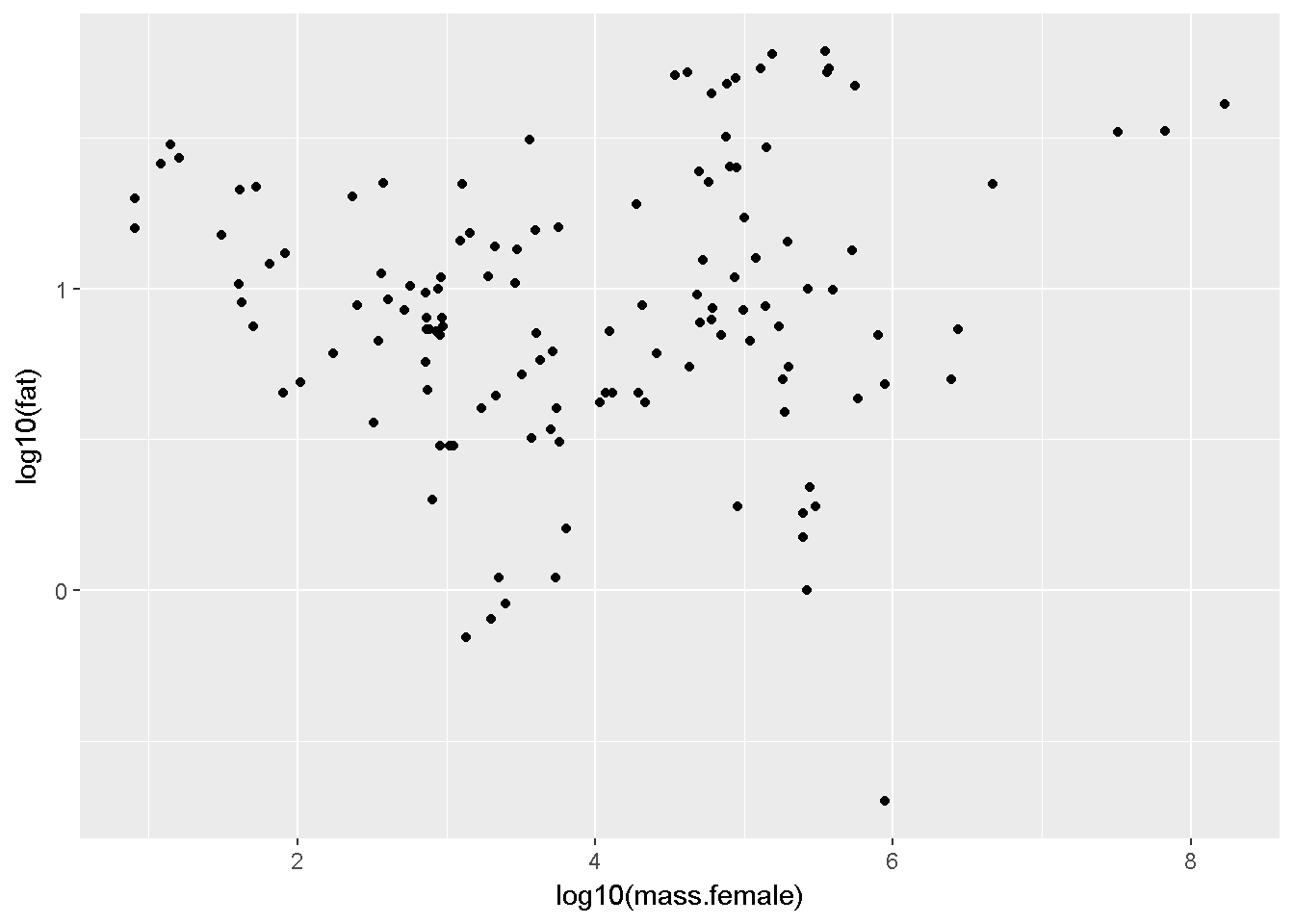Log transform x, logit transform y variable • The data are percentages and therefore the logit is a more appropriate transformation • The car package has a logit() command • car::logit() can accept proportions ([0,1]) or percentages ([0,100]) • the fat data are percentages so we use the arguement “percents = TRUE”" library(car) qplot(y = logit(fat, percents = T), x = log10(mass.female), data = dat2)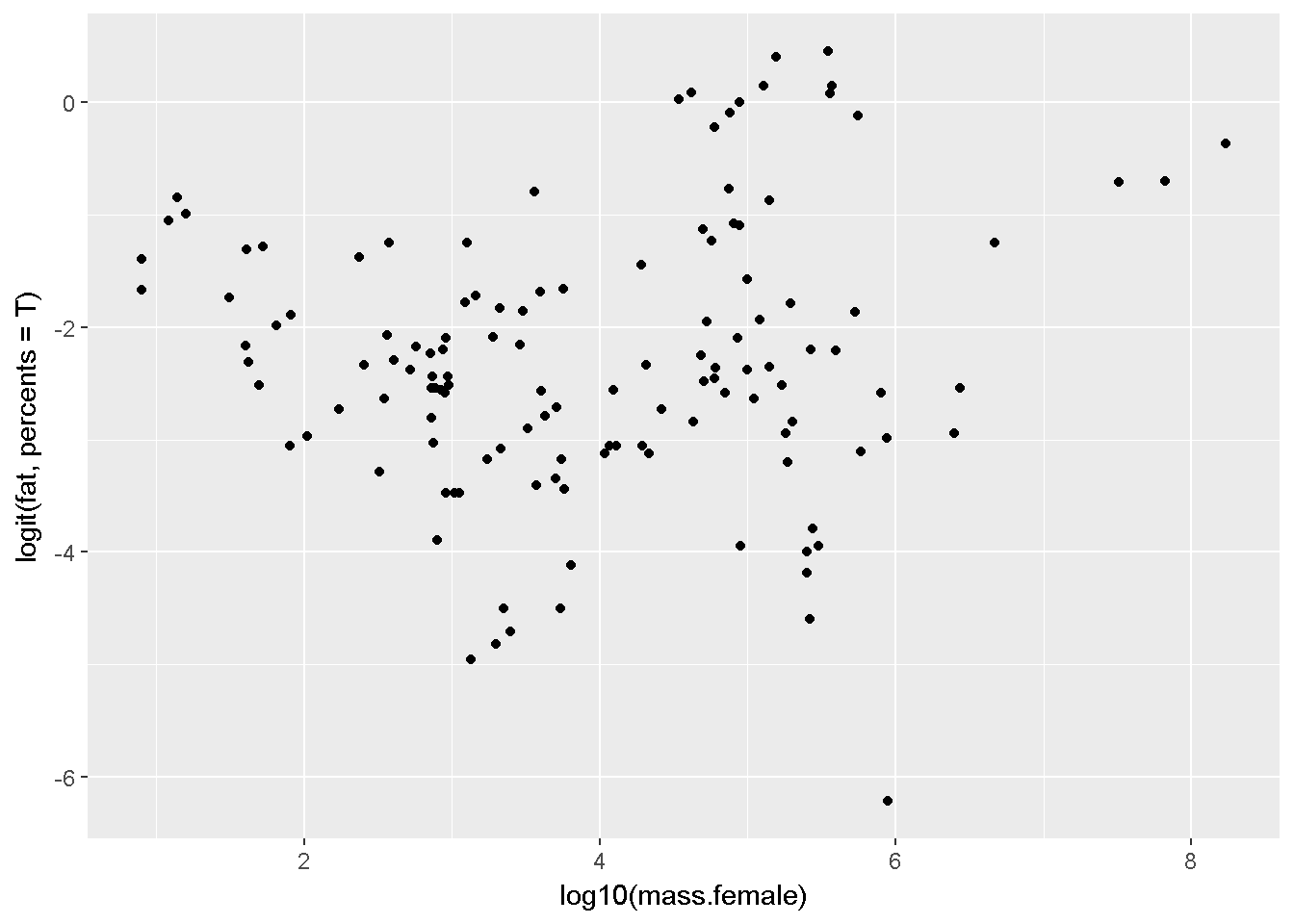Hard code transformation To make code cleaner make new columns with the transformed data #x variable fat ## The authors' transformation of the dat2$fat.log10 <- log10(dat2$fat) ## The logit transformation dat2$fat.logit <-logit(dat2$fat, percents = T) #y variable female mass dat2$mass.log10 <- log10(dat2$mass.female) Colors library(car) qplot(y = fat.log10, x = mass.log10, data = dat2, color = Australia)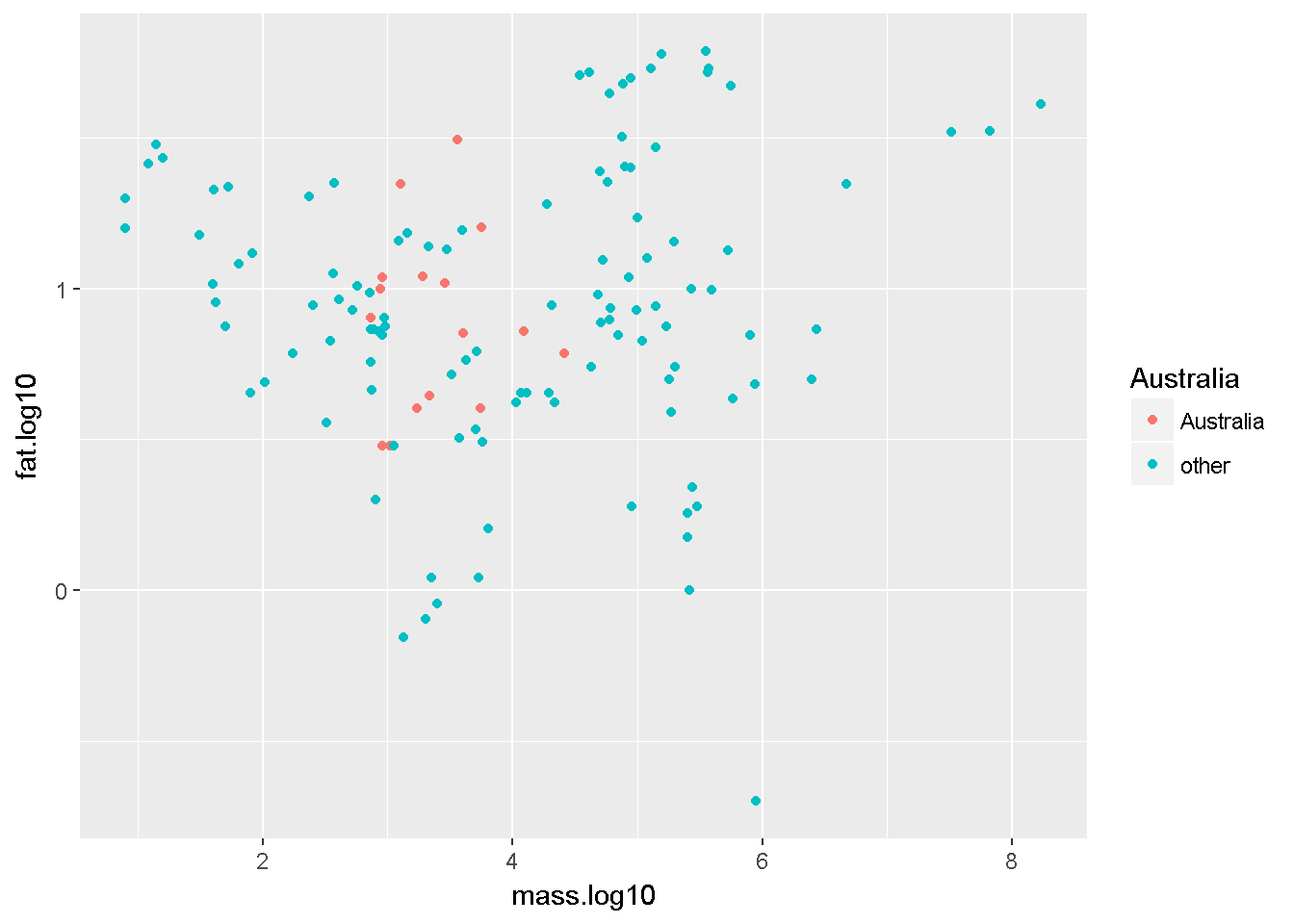Shape library(car) qplot(y = fat.log10, x = mass.log10, data = dat2, shape = diet)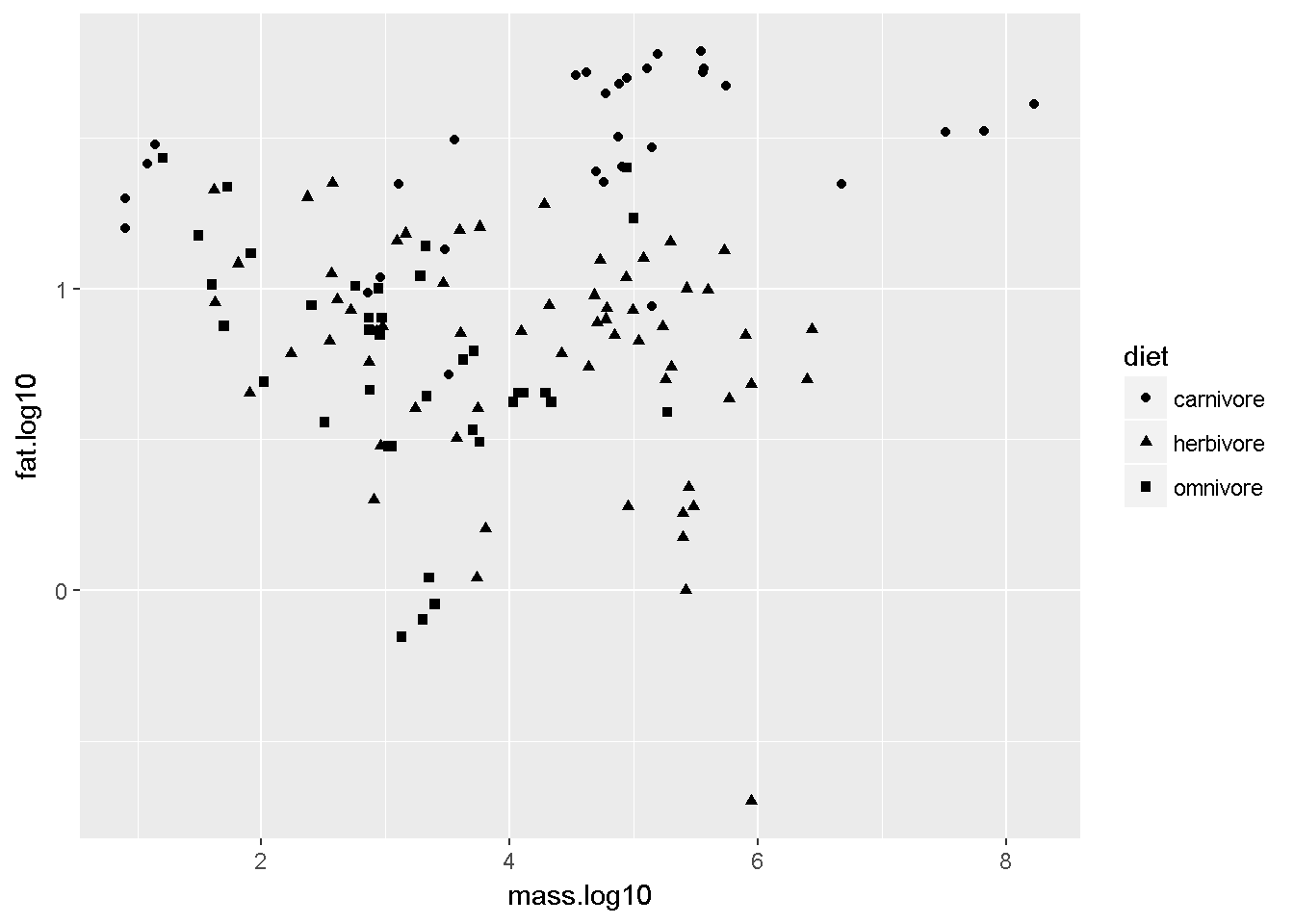Colors and shape library(car) qplot(y = fat.log10, x = mass.log10, data = dat2, color = Australia, shape = diet)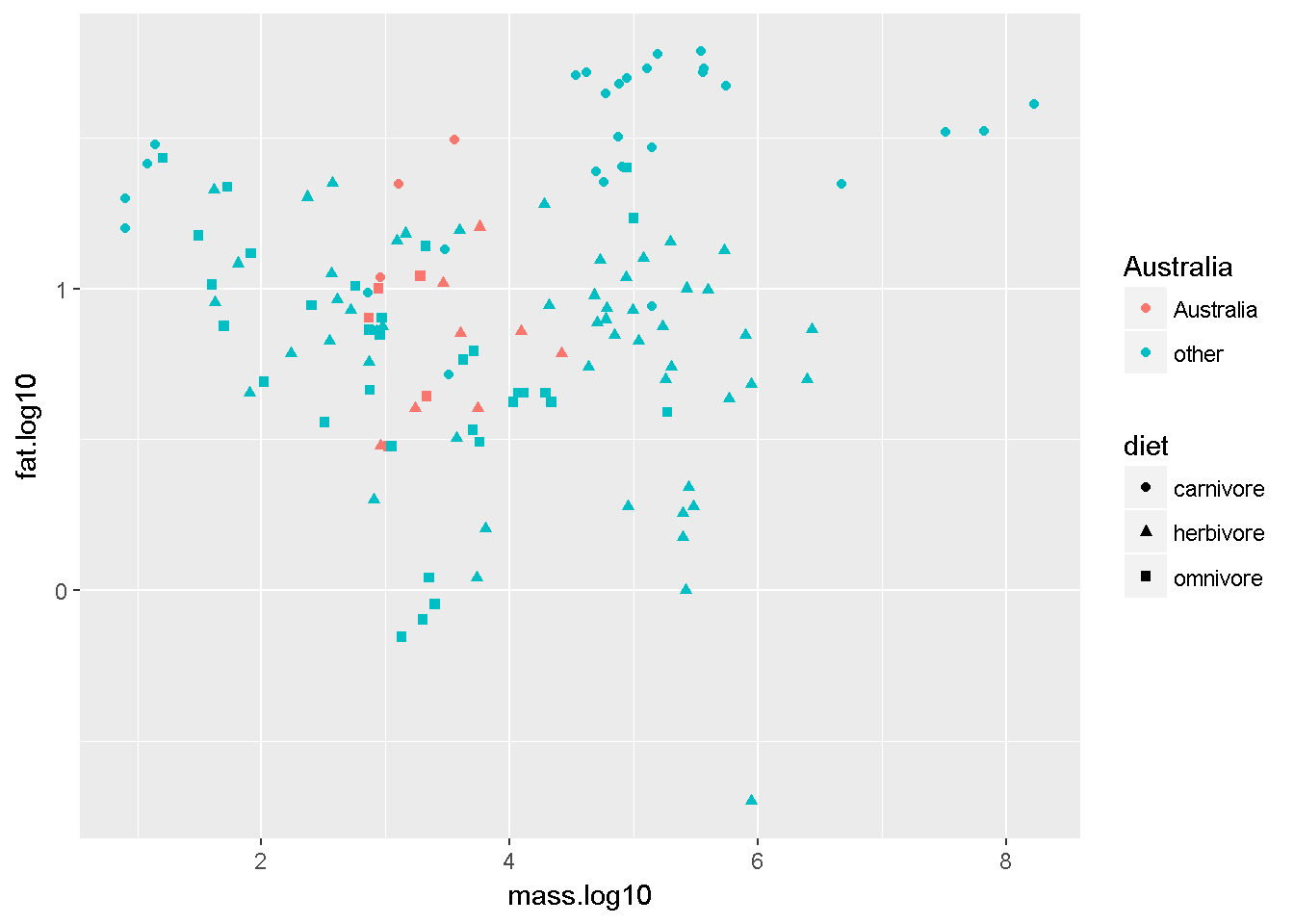Facets Plot different groups on sep. panels. This allows you to explore structure of data. This reveals something interesting - aquatic carnivores seem to have a positive fat~mass relationship, while terrestrial carnivores seem to have a negative one. This is interesting, since the authors found no overal significant relationship between body size and milk composition, but they did not explore any interactions. This would be difficult to model with the given sample size though b/c it imlies a massdietaquatic interaction. Since all of the aquatic animals in the dataset are carnivores, the carnivore diet category could be split into terrestrial and aquatic groups. qplot(y = fat.log10, x = mass.log10, data = dat2, color = Australia, shape = diet, facets = biome ~ diet)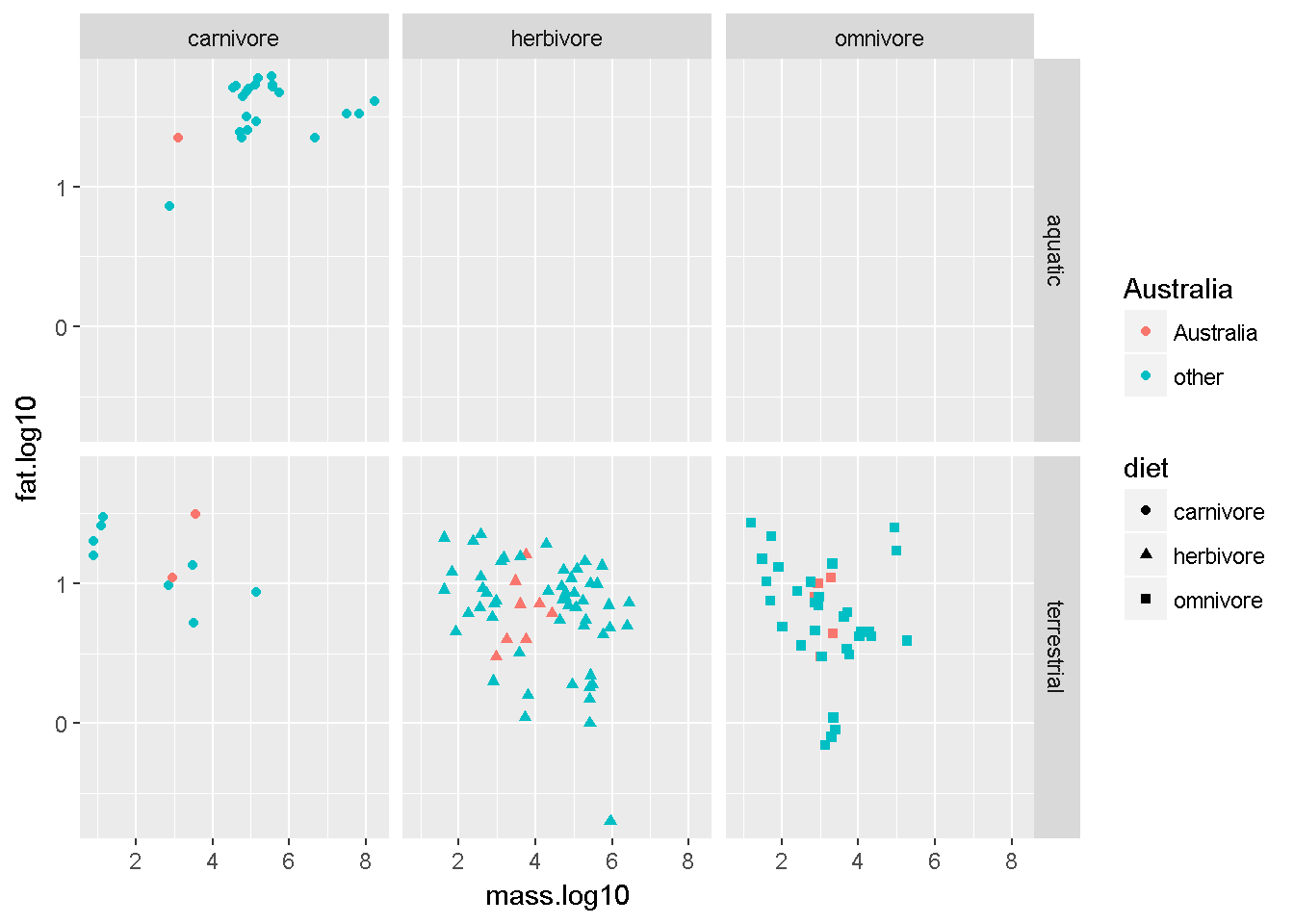Note the outliers the hand off the bottom of two of the three lower panels. I’ll use colors to see what they are qplot(y = fat.log10, x = mass.log10, data = dat2, color = order, shape = diet, facets = biome ~ diet)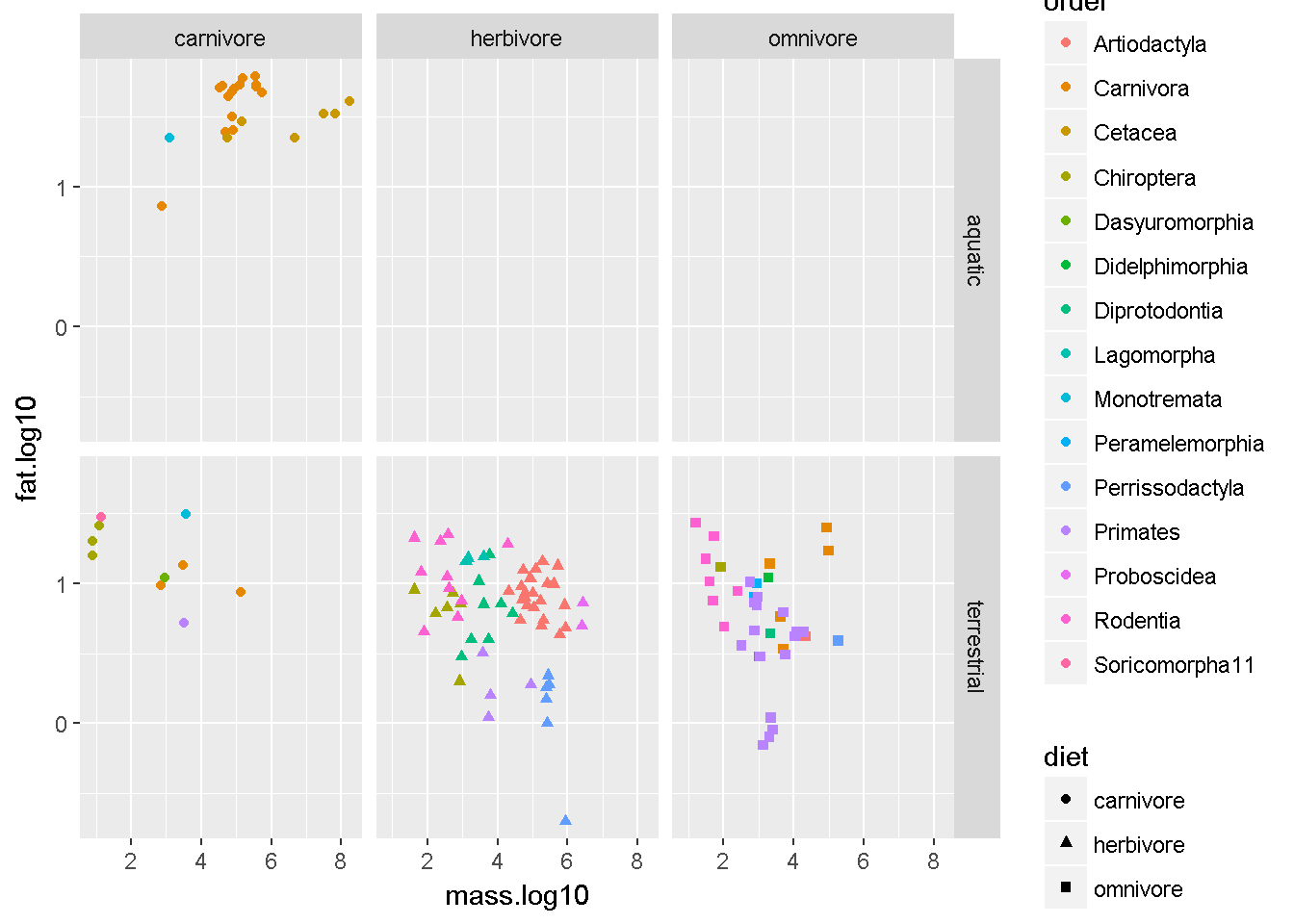In the herbivore-terrestrial panel it appears like Perrissodactyla all form a line. These are odd-toed ungulates like rhinos and horses. The really low triangle I believe is a rhino. IN the Omnivore-terrestrial panel it looks like clump of primates are outliers. I’m going to guess these are lemurs. qplot(y = fat.log10, x = mass.log10, data = dat2, color = family, shape = diet, facets = biome ~ diet)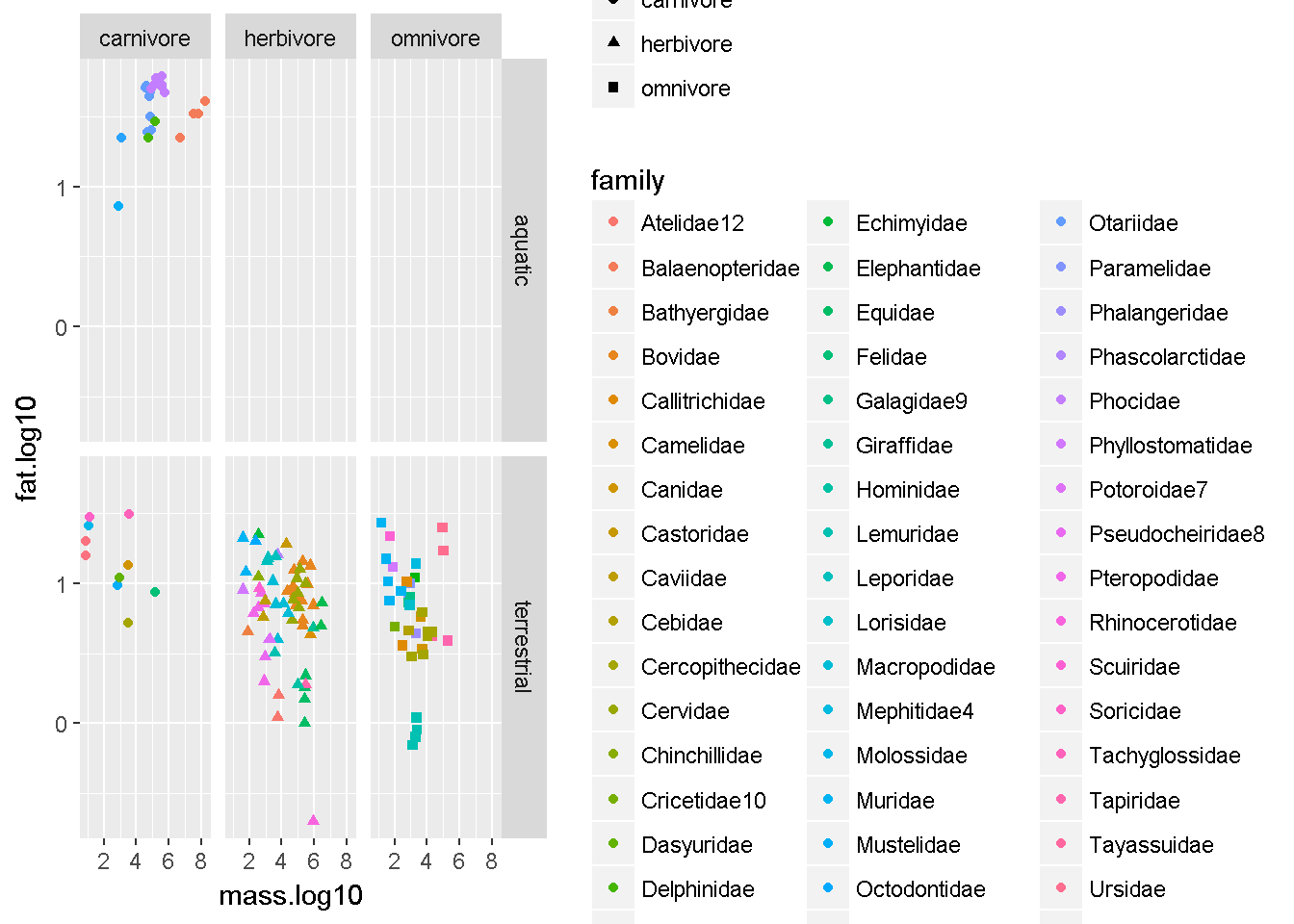Make an index for lemurs i.lemur <- which(dat2$family == "Lemuridae")

dat2$lemurs <- "not.a.lemur" dat2$lemurs[i.lemur] <- "lemur"

Yup, its the lemurs. This indicates that for their size they have really lean milk (low fat)

qplot(y = fat.log10,
x = mass.log10,
data = dat2,
color = lemurs,
shape = diet,
facets = biome ~ diet)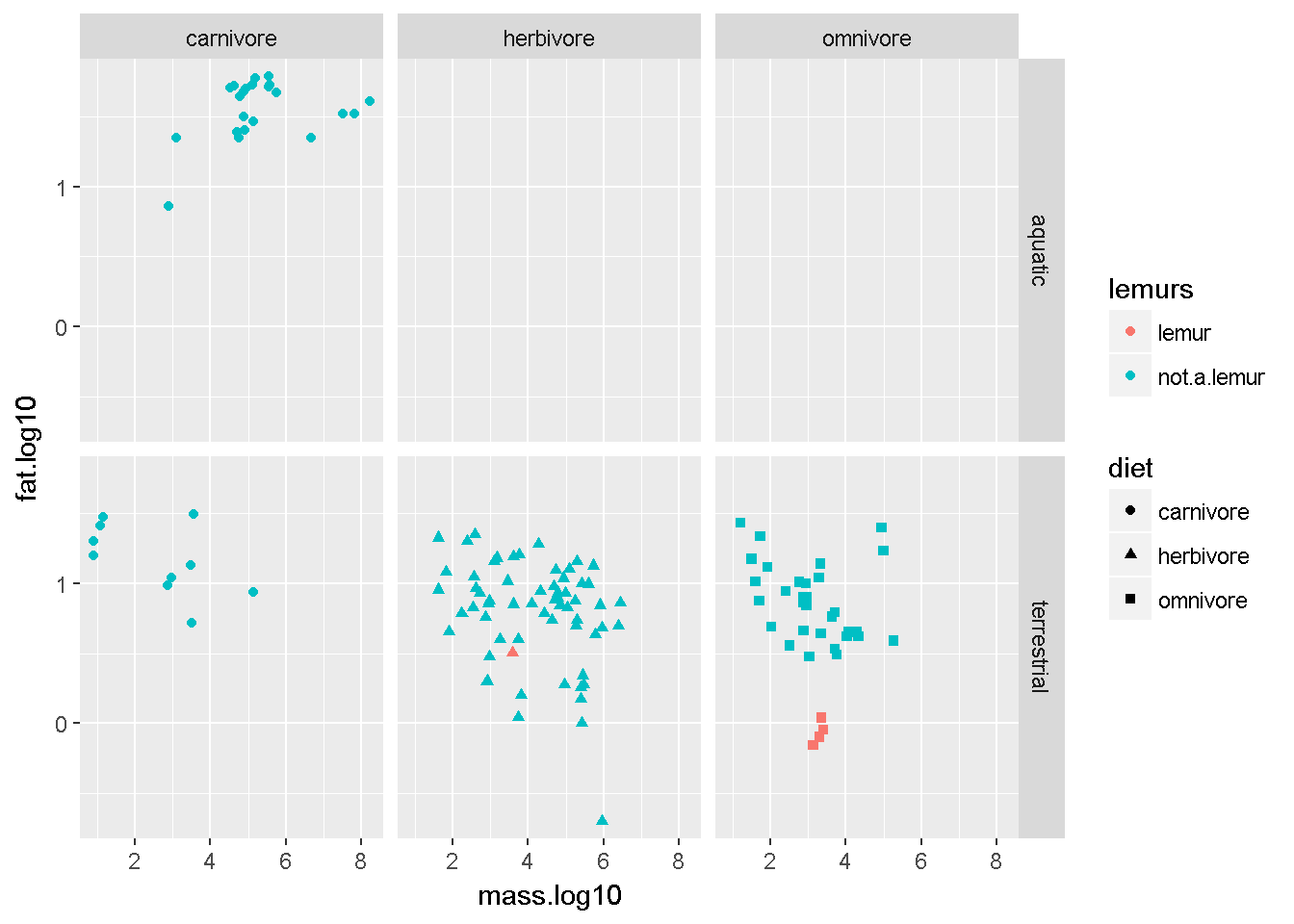Save data

Save our data subset

write.csv(dat2,"milk_subset.csv")